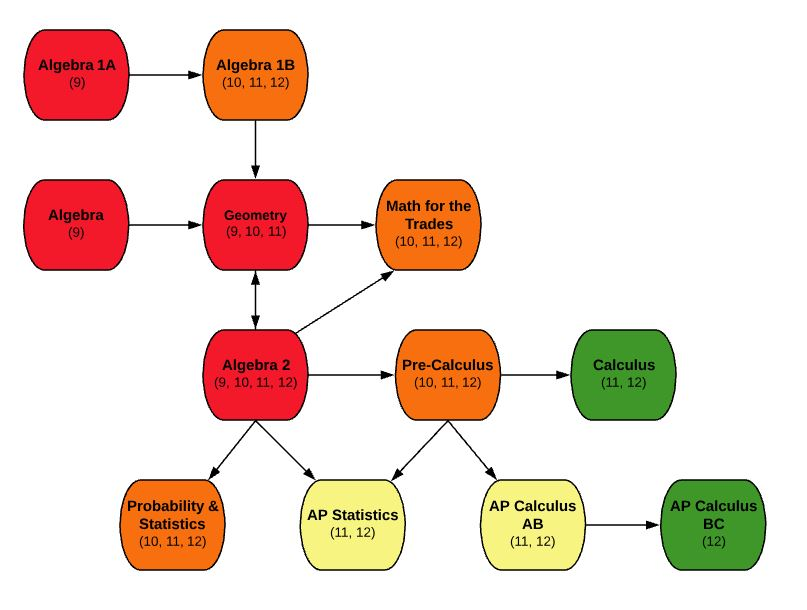EDIT MAIN

# MA110 Algebra9

Mathematics

Standard

1

This is a year long course that requires selection of both semesters: S1 and S2. Gateway Technical College””Pre-Algebra or successful completion of 8th grade mathematics””. Provide students with a basis for advanced mathematics and aid them in solving mathematical problems. The course uses modern terminology as it applies to Algebra. Topics covered are: one- and two-step percent word problems, percent change, average, simple probability, counting techniques in probability, place value, number lines and the coordinate plane, multi-step equations, parallel lines, area and perimeter, unit conversion, representing data in multiple ways, pattern identification, manipulation of algebraic expressions, slope and linear graphing, angle properties, area of geometric figures, and function notation. The course focuses on the 400 level problems and extends into the 500 level problems for the ACT College Readiness Standards. A scientific calculator is required for this course (we recommend the TI30XIIS or graphing calculator TI84).

• Evaluate expressions using properties and the order of operations
• Solve complex linear equations
• Write and graph linear equations in different forms including standard form and slope intercept form
• Simplify polynomial expressions by using properties of exponents
• Solve systems of equations by using graphing, substitution, and elimination methods
• Solve and graph one-step inequalities
• Factor polynomial expressions